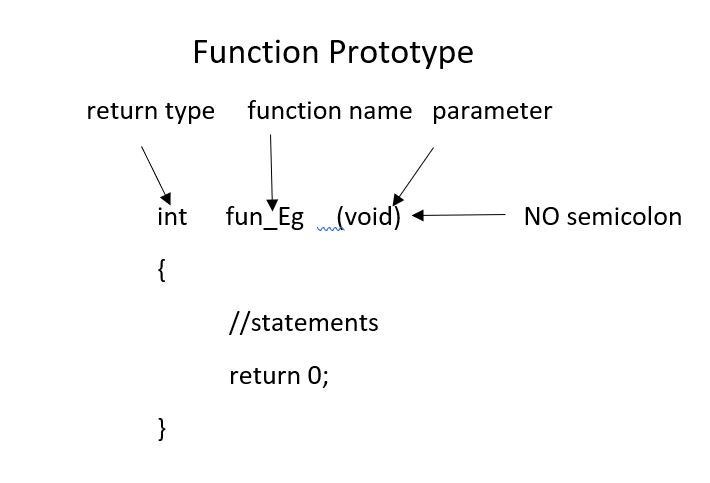# Functions in C

#### Software Engineering function C C++Get FREE domain for 1st year and build your brand new site

Reading time: 20 minutes | Coding time: 2 minutes

A function is a set of statements that take inputs, do some specific computation and produces output.

The idea is to put some commonly or repeatedly done task together and make a function, so that instead of writing the same code again and again for different inputs, we can call the function.

### Function Declaration

Function declaration tells compiler about number of parameters function takes, data-types of parameters and return type of function. Putting parameter names in function declaration is optional in function declaration, but it is necessary to put them in definition. Below are example of function declarations. (parameter names are not there in below declarations).// A function that takes two integers as parameters
// and returns an integer
int max(int, int);

// A function that takes a int pointer and an int variable as parameters
// and returns an integer of type int
int *swap(int*,int);

// A function that takes a charas parameters
// and returns an reference variable
char &call(char b);

// A function that takes a char and an int as parameters
// and returns an integer
int fun(char, int);


It is always recommended to declare a function before it is used.

In C, we can do both declaration and definition at same place.

C also allows to declare and define functions separately, this is specially needed in case of library functions. The library functions are declared in header files and defined in library files. Below is an example declaration.

### Parameter Passing to functions

The parameters passed to function are called actual parameters. For example, in the above program 10 and 20 are actual parameters.
The parameters received by function are called formal parameters. For example, in the above program x and y are formal parameters.

There are two most popular ways to pass parameters namely:

• Pass by value
• Pass by reference

Pass by Value In this parameter passing method, values of actual parameters are copied to function’s formal parameters and the two types of parameters are stored in different memory locations. So any changes made inside functions are not reflected in actual parameters of caller.

Pass by Reference Both actual and formal parameters refer to same locations, so any changes made inside the function are actually reflected in actual parameters of caller.

In C, parameters are always passed by value. Parameters are always passed by value in C. For example. in the below code, value of x is not modified using the function fun().

#include <stdio.h>
void fun(int x)
{
x = 30;
}

int main(void)
{
int x = 20;
fun(x);
printf("x = %d", x);
return 0;
}


Output:

    x = 20


However, in C, we can use pointers to get the effect of pass by reference. For example, consider the below program. The function fun() expects a pointer ptr to an integer (or an address of an integer). It modifies the value at the address ptr. The dereference operator * is used to access the value at an address. In the statement ‘*ptr = 30’, value at address ptr is changed to 30. The address operator & is used to get the address of a variable of any data type. In the function call statement ‘fun(&x)’, the address of x is passed so that x can be modified using its address.

# include <stdio.h>
void fun(int *ptr)
{
*ptr = 30;
}

int main()
{
int x = 20;
fun(&x);
printf("x = %d", x);

return 0;
}


Output:

    x = 30


### Important points about functions in C

1. Every C program has a function called main() that is called by operating system when a user runs the program.

2. Every function has a return type. If a function doesn’t return any value, then void is used as return type.Moreover if the return type of the function is void ,we still can use return statement in the body of function definition by not specifying any constant,variable,etc. with it ,by only mentioning the ‘return;’ statement which would symbolise the termination of the function as shown below:

void function name(int a)
{
.......  //Function Body
return;  //Function execution would get terminated
}

1. In C, functions can return any type except arrays and functions. We can get around this limitation by returning pointer to array or pointer to function.

2. Empty parameter list in C mean that the parameter list is not specified and function can be called with any parameters. In C, it is not a good idea to declare a function like fun(). To declare a function that can only be called without any parameter, we should use:

void fun(void)


As a side note, in C++, empty list means function can only be called without any parameter. In C++, both void fun() and void fun(void) are same.

1. If in a C program, a function is called before its declaration then the C compiler automatically assumes the declaration of that function in the following way:
int function name();


In that case, if the return type of that function is different than INT ,compiler would show an error.

### Question

#### In C, function parameters are always

Passed by value
Passed by reference
Passed by value result
Depends of variable type
In C, function parameters are always passed by value. Pass-by-reference is simulated in C by explicitly passing pointer values.

Read about Recursion which is another way of implementing loops using functions#### Harshita Sahai

Maintainer at OpenGenus | Previously Software Developer, Intern at OpenGenus (June to August 2019) | B.Tech in Information Technology from Guru Gobind Singh Indraprastha University (2017 to 2021)